﻿ 基于无语音概率的语音增强算法 Speech Enhancement Algorithm Combining Speech Absence Probability

Hans Journal of Wireless Communications
Vol.08 No.04(2018), Article ID:26130,7 pages
10.12677/HJWC.2018.84016

Speech Enhancement Algorithm Combining Speech Absence Probability

Ruirui Han, Ying Gao, Chen Chen

School of Opto-Electronic Information, Yantai University, Yantai Shandong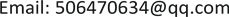Received: Jul. 1st, 2018; accepted: Jul. 18th, 2018; published: Jul. 30th, 2018ABSTRACT

The research work of this paper is mainly on the basis of the amplitude squared spectrum least mean square estimator and proposes a new algorithm. Due to the uncertainty of the speech in the statistical model of noisy speech, the unified processing of speech signals will inevitably result in the loss of speech components, which will affect the performance of speech enhancement. Therefore, this paper mainly studies and estimates the frequency of each signal. The speech probability is then combined with the gain function of the squared spectrum least mean square error algorithm to derive a new gain function. Finally, we can see through the experimental simulation, the algorithm proposed in this paper can significantly improve the voice quality and improve the intelligibility of the voice.

Keywords:Speech Enhancement, Speech Absence Probability, Minimum Mean-Squared Error, Gain Function1. 引言

2. 语音增强算法基本理论

${Y}_{k}^{2}={X}_{k}^{2}+{D}_{k}^{2}$ (1)

${\stackrel{^}{X}}_{k}^{2}=E\left\{{X}_{k}^{2}|{Y}_{k}^{2}\right\}={\int }_{0}^{{Y}_{k}^{2}}{X}_{k}^{2}f\left({X}_{k}^{2}|{Y}_{k}^{2}\right)\text{d}{X}_{k}^{2}$ (2)

$f\left({X}_{k}^{2}|{Y}_{k}^{2}\right)=\frac{f\left({Y}_{k}^{2}|{X}_{k}^{2}\right)f\left({X}_{k}^{2}\right)}{f\left({Y}_{k}^{2}\right)}=\left\{\begin{array}{l}{\Psi }_{k}\mathrm{exp}\left(-\frac{{X}_{k}^{2}}{\lambda \left(k\right)}\right)\text{\hspace{0.17em}}\text{\hspace{0.17em}}\text{\hspace{0.17em}}\text{\hspace{0.17em}}\text{\hspace{0.17em}}\text{\hspace{0.17em}}{\sigma }_{x}^{2}\ne {\sigma }_{d}^{2}\\ \frac{1}{{Y}_{k}^{2}}\text{\hspace{0.17em}}\text{\hspace{0.17em}}\text{\hspace{0.17em}}\text{\hspace{0.17em}}\text{\hspace{0.17em}}\text{\hspace{0.17em}}\text{\hspace{0.17em}}\text{\hspace{0.17em}}\text{\hspace{0.17em}}\text{\hspace{0.17em}}\text{\hspace{0.17em}}\text{\hspace{0.17em}}\text{\hspace{0.17em}}\text{\hspace{0.17em}}\text{\hspace{0.17em}}\text{\hspace{0.17em}}\text{\hspace{0.17em}}\text{\hspace{0.17em}}\text{\hspace{0.17em}}\text{\hspace{0.17em}}\text{\hspace{0.17em}}\text{ }\text{ }\text{\hspace{0.17em}}\text{\hspace{0.17em}}\text{\hspace{0.17em}}\text{\hspace{0.17em}}{\sigma }_{x}^{2}={\sigma }_{d}^{2}\end{array}$ (3)

$\frac{1}{\lambda \left(k\right)}=\frac{1}{{\sigma }_{x}^{2}\left(k\right)}-\frac{1}{{\sigma }_{x}^{2}\left(k\right)}$ (4)

${\Psi }_{k}=\frac{1}{\lambda \left(k\right)\left\{1-\mathrm{exp}\left[-\frac{{Y}_{k}^{2}}{\lambda \left(k\right)}\right]\right\}}$ (5)

${X}_{k}^{2}=\left\{\begin{array}{l}\left(\frac{1}{{v}_{k}}-\frac{1}{\mathrm{exp}\left({v}_{k}\right)-1}\right){Y}_{k}^{2},\text{\hspace{0.17em}}\text{\hspace{0.17em}}{\sigma }_{x}^{2}\ne {\sigma }_{d}^{2}\\ \frac{1}{2}{Y}_{k}^{2},\text{ }\text{ }\text{ }\text{ }\text{ }\text{ }\text{ }\text{ }\text{ }\text{ }\text{ }\text{ }\text{ }\text{ }\text{ }\text{ }\text{ }\text{ }\text{ }\text{ }\text{ }\text{ }\text{ }\text{ }\text{ }\text{ }\text{ }\text{ }\text{ }\text{ }\text{ }\text{ }\text{ }\text{ }\text{ }\text{ }\text{ }\text{ }\text{ }\text{ }\text{ }\text{ }\text{ }\text{ }\text{ }\text{ }\text{ }\text{ }\text{ }\text{ }\text{ }\text{ }\text{ }\text{ }\text{ }\text{ }\text{ }\text{ }\text{ }\text{ }\text{ }\text{\hspace{0.17em}}\text{ }\text{ }\text{ }\text{ }\text{ }\text{ }\text{ }\text{ }\text{ }\text{ }{\sigma }_{x}^{2}={\sigma }_{d}^{2}\end{array}$ (6)

${G}_{\text{MMSE-MSS}}=\left\{\begin{array}{l}\sqrt{\frac{1}{{v}_{k}}-\frac{1}{\mathrm{exp}\left({v}_{k}\right)-1}},\text{ }\text{ }\text{ }\text{ }\text{ }\text{ }\text{ }\text{ }\text{ }\text{ }\text{ }\text{ }\text{ }\text{ }\text{ }\text{\hspace{0.17em}}{\sigma }_{x}^{2}\ne {\sigma }_{d}^{2}\\ \sqrt{\frac{1}{2},}\text{ }\text{ }\text{ }\text{ }\text{ }\text{ }\text{ }\text{ }\text{ }\text{ }\text{ }\text{ }\text{ }\text{ }\text{ }\text{ }\text{ }\text{ }\text{ }\text{ }\text{ }\text{ }\text{ }\text{ }\text{ }\text{ }\text{ }\text{ }\text{ }\text{ }\text{ }\text{ }\text{ }\text{ }\text{ }\text{ }\text{ }\text{ }\text{ }\text{ }\text{ }\text{ }\text{ }\text{ }\text{ }\text{ }\text{ }\text{ }\text{ }\text{ }\text{ }\text{ }\text{ }\text{ }\text{ }\text{ }\text{ }\text{ }\text{ }\text{ }\text{ }\text{ }\text{ }\text{ }\text{ }\text{ }\text{ }\text{ }\text{ }\text{ }\text{ }\text{ }\text{ }\text{ }\text{ }\text{ }{\sigma }_{x}^{2}={\sigma }_{d}^{2}\end{array}$ (7)

3. 本文提出的融合无语音概率的语音增强算法

${f}_{{X}_{k}^{2}}\left({X}_{k}^{2}\right)=\frac{1}{{\sigma }_{x}^{2}}\mathrm{exp}\left[-\frac{{X}_{k}^{2}}{{\sigma }_{x}^{2}}\right]$ (8)

${f}_{{D}_{k}^{2}}\left({D}_{k}^{2}\right)=\frac{1}{{\sigma }_{d}^{2}}\mathrm{exp}\left[-\frac{{D}_{k}^{2}}{{\sigma }_{d}^{2}}\right]$ (9)

${f}_{{Y}_{k}^{2}}\left({Y}_{k}^{2}\right)=\frac{1}{{\sigma }_{x}^{2}-{\sigma }_{d}^{2}}\left(\mathrm{exp}\left(-\frac{{Y}_{k}^{2}}{{\sigma }_{x}^{2}}\right)-\mathrm{exp}\left(-\frac{{Y}_{k}^{2}}{{\sigma }_{d}^{2}}\right)\right)$ (10)

$\left\{\begin{array}{l}{H}_{0}^{k}:{Y}_{k}^{2}={D}_{k}^{2}\\ {H}_{1}^{k}:{Y}_{k}^{2}={X}_{k}^{2}+{D}_{k}^{2}\end{array}$ (11)

$P\left({Y}_{k}^{2}|{H}_{1}^{k}\right)=\frac{1}{{\sigma }_{x}^{2}-{\sigma }_{d}^{2}}\left(\mathrm{exp}\left(-\frac{{Y}_{k}^{2}}{{\sigma }_{x}^{2}}\right)-\mathrm{exp}\left(-\frac{{Y}_{k}^{2}}{{\sigma }_{d}^{2}}\right)\right)$ (12)

$P\left({Y}_{k}^{2}|{H}_{0}^{k}\right)=\frac{1}{{\sigma }_{d}^{2}}\mathrm{exp}\left(-\frac{{Y}_{k}^{2}}{{\sigma }_{d}^{2}}\right)$ (13)

$P\left({Y}_{k}^{2}|{H}_{1}^{k}\right)=\frac{P\left({Y}_{k}^{2}|{H}_{1}^{k}\right)P\left({H}_{1}^{k}\right)}{P\left({Y}_{k}^{2}|{H}_{0}^{k}\right)P\left({H}_{0}^{k}\right)+P\left({Y}_{k}^{2}|{H}_{1}^{k}\right)P\left({H}_{1}^{k}\right)}=\frac{\Lambda \left({Y}^{2}\right)}{1+\Lambda \left({Y}^{2}\right)}=G$ (14)

${G}_{\text{new}}={G}_{\text{MMSE-MSS}}\cdot \sqrt{G}$ (15)

${\stackrel{^}{X}}_{k}^{2}={G}_{\text{new}}\cdot {Y}_{k}^{2}$ (16)

4. 仿真实验结果分析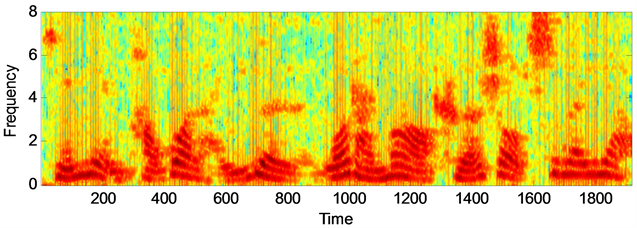(a) 纯净语音信号语谱图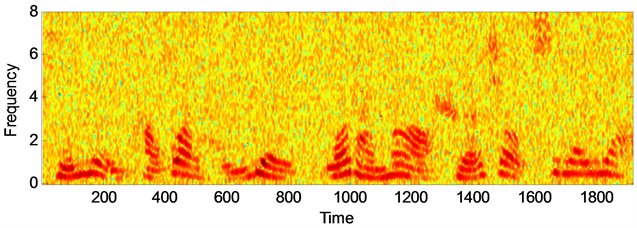(b) 带噪语音信号语谱图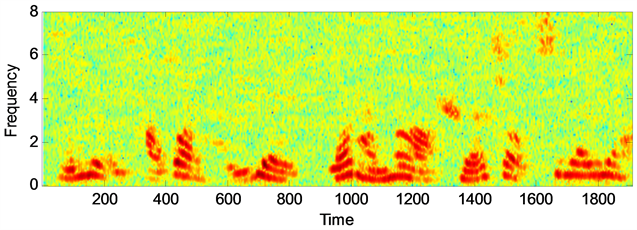(c) 基于MMSE-MSS算法的增强语音语谱图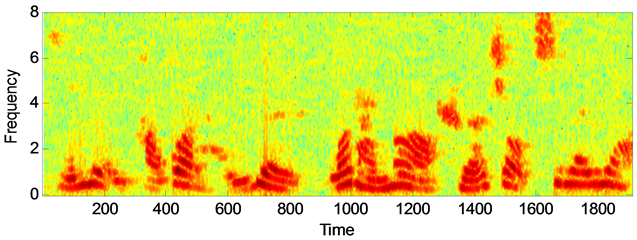(d) 基于本文算法的增强语音信号语谱图

Figure 1. The spectrum of speech signal of different algorithms under white noise. (SNR = 10 dB)Table 1. The data comparison table of the two algorithms

5. 小结

Speech Enhancement Algorithm Combining Speech Absence Probability[J]. 无线通信, 2018, 08(04): 141-147. https://doi.org/10.12677/HJWC.2018.84016

1. 1. Lu, Y. and Loizou, P.C. (2011) Estimators of the Magnitude-Squared Spectrum and Methods for Incorporating SNR Uncertainty. IEEE Transactions on Audio, Speech, and Language Processing, 19, 1123-1137.
https://doi.org/10.1109/TASL.2010.2082531

2. 2. Diethom, E.J. (2000) Subband Noise Reduction Methods for Speech Enhancement. Acoustic Signal Processing for Telecommunication. Springer US, New York, 155-178.

3. 3. Xia, B. and Bao, C. (2014) Wiener Filtering Based Speech Enhancement with Weighted Denoising Auto-Encoder and Noise Classification. Speech Communication, 60, 13-29.
https://doi.org/10.1016/j.specom.2014.02.001

4. 4. 卢志强. 基于新型先验信噪比估计的语音增强算法的对比研究[D]: [硕士学位论文]. 长沙: 湖南大学.

5. 5. Papoulis, A. and Pillai, S.U. (2002) Probability, Random Variables and Stochastic Processes with Errata Sheet. McGraw-Hill Education, New York, 31.

6. 6. Huan, Z. (2014) A New Soft Masking Method for Speech Enhancement in the Frequency Domain. Elektronika ir Elektrotechnika, 20, 1392-1215.

7. 7. Cohen, I. (2003) Noise Spectrum Estimation in Adverse Environments: Improved Minima Contorlled Recursive Averraging. IEEE Transaction on Speech and Audio Processing, 11, 466-475.
https://doi.org/10.1109/TSA.2003.811544

8. 8. Ephraim, Y. and Malah, D. (1984) Speech Enhancement Using a Minimum-Mean Squared Error Short-Time Spectral Amplitude Estimator. IEEE Transactions on Acoustics, Speech and Signal Processing, 32, 1109-1121.
https://doi.org/10.1109/TASSP.1984.1164453

9. 9. Taal, C.H., Hendriks, R.C., Heusdens, R., et al. (2011) An Algorithm for Intelligibility Prediction of Time-Frequency Weighted Noisy Speech. IEEE Transactions on Audio Speech & Language Processing, 19, 2125-2136.
https://doi.org/10.1109/TASL.2011.2114881

10. 10. Abramson, A. and Cohen, I. (2010) Simultaneous Detection and Estimation Approach for Speech Enhancement. IEEE Transactions on Audio Speech & Language Processing, 15, 2348-2359.
https://doi.org/10.1109/TASL.2007.904231